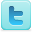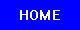Gravitation & Gravity
Science Fair Projects
Ideas and Sample Projects by Field

Center of Gravity at Work

 Physics P=Project   E=Experiment Research and explain how scientists detected, at LIGO, gravitational waves that were predicted by Einstein’s Theory of General Relativity. [E] [E] [E] [E] Find out if the diameter of a PVC pipe would affect the speed of a small metal ball travelling inside the pipe - explore the relationship of friction and gravity inside the pipe. [E] Bending space-time, matter and gravity [E] Build an accelerometer and use it to measure acceleration and gravity-induced reaction forces on a roller-coaster ride. [E] Prove Isaac Newton's laws of gravity and Albert Einstein's General Theory of Relativity. [E] Proving Isaac Newton's Universal Gravitation by Warping Space-Time [E] Repeat Eötvös experiment (the correlation between inertial mass and gravitational mass, demonstrating that the two were one and the same) [E] [E] Relativity and Black Holes Engineering Build a gravity-powered sorting machine that can separate small and large plastic spheres. [E] Determine the effects of aft weight and nose weight (center of gravity) on paper airplanes. [E] Using Simple Models to Predict the Effects of Gravity on Projectiles [E] How Does Center of Gravity Affect a Crane Design? [E] Will the increasing of the distance between the center of gravity (CG) and the center of wind pressure (CP) increase the rocket's stability and performance of flight [E] Astronomy Using Magnetic Levitation to determine whether it is possible to measure Gravity using the moon's gravitational pull. [P] Measuring the Speed of Gravity using Ocean Tide Models in Conjunction with Solar and Lunar Position Tracks [E] Botany Tropism Earth Sciences Finding Your Location Using Gravity, the Earth's Magnetic Field, and a Sundial [E] Medicine and Human Anatomy Explore how changing your center of gravity affects how well you can balance. [E] Solar & Renewable Energy Determine whether it is possible to harness wasted gravitational potential energy and convert it into easily accessed kinetic energy. Application of this principle is a garbage can that can bring itself out to the curb when full and back to the house when empty. [E] Useful Links R=Resource Science Fair Projects Resources [R] Science Fair Projects A-Z [R] Citation Guides, Style Manuals, Reference [R] Safety Resources [R] [R] Science Fair Books

 Science Fair Project Guide Home Science Fair Project Types The Scientific Method - How to Experiment The Display Board Topics, Ideas, Sample Projects

Follow Us On:Privacy Policy - Site Map - About Us - Letters to the Editor

Comments and inquiries:
webmaster@julianTrubin.comLast updated: January 2017
Copyright © 2003-2017 Julian Rubin